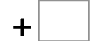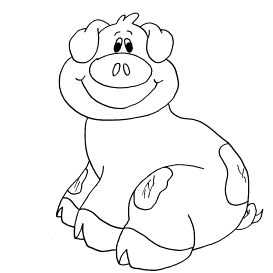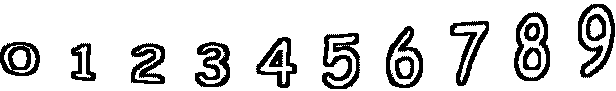5= 12   0= 63= 8   0= 8   3= 9   1= 8 1= 11   2= 9   3= 5   1= 4